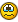Recommended Posts

Hi guys,

In one of my scripts I've to generate 5 numbers that are ALL differents.

I've tried to do something like that :

Do

\$p = Random(1, 10, 1)
\$p1 = Random(1, 10, 1)
\$p2 = Random(1, 10, 1)
\$p3 = Random(1, 10, 1)
\$p4 = Random(1, 10, 1)

Until (\$p <> \$p1 <> \$p2 <> \$p3 <> \$p4)

But it doesn't work.

The only way I've found is to do all the testslike this :

Do

\$p = Random(1, 10, 1)
\$p1 = Random(1, 10, 1)
\$p2 = Random(1, 10, 1)
\$p3 = Random(1, 10, 1)
\$p4 = Random(1, 10, 1)
Until (\$p <> \$p1 And \$p <> \$p2 And \$p <> \$p2 And \$p <> \$p3 And \$p <> \$p4 And \$p1 <> \$p2 And \$p1 <> \$p3 And \$p1 <> \$p4 And \$p2 <> \$p3 And \$p2 <> \$p4 And \$p3 <> \$p4)

... imagine if I've to generate 50 numbers between 1 to 1000, the test should be difficult to write!

It's there a best way to do this ???

Edited by akorx

AkorxMail akorx@yahoo.fr

Share on other sites

Hi,

There are multiple possibilities using an array.

One of them is to fill the array with numbers from 1 to 10 (or other limits) and then scramble it.

Br, FireFox.

OS : Win XP SP2 (32 bits) / Win 7 SP1 (64 bits) / Win 8 (64 bits) | Autoit version: latest stable / beta.
Hardware : Intel(R) Core(TM) i5-2400 CPU @ 3.10Ghz / 8 GiB RAM DDR3.

My UDFs : Skype UDF | TrayIconEx UDF | GUI Panel UDF | Excel XML UDF | Is_Pressed_UDF

My Projects : YouTube Multi-downloader | FTP Easy-UP | Lock'n | WinKill | AVICapture | Skype TM | Tap Maker | ShellNew | Scriptner | Const Replacer | FT_Pocket | Chrome theme maker

My Examples : Capture toolIP Camera | Crosshair | Draw Captured Region | Picture Screensaver | Jscreenfix | Drivetemp | Picture viewer

My Snippets : Basic TCP | Systray_GetIconIndex | Intercept End task | Winpcap various | Advanced HotKeySet | Transparent Edit control

Share on other sites

Try this one#include <array.au3>
Global \$RandArray
\$i = 0

While \$i < 50
\$num = Random(1, 100, 1)
_ArraySearch(\$RandArray, \$num)
If @error Then
\$RandArray[\$i] = \$num
\$i += 1
EndIf
WEnd

_ArrayDisplay(\$RandArray)

Share on other sites

#include <Array.au3>

Local \$p, \$n
For \$i = 0 to UBound(\$p)-1
Do
\$n = Random(1, 10, 1)
Until  _ArraySearch(\$p, \$n) = -1
\$p[\$i] = \$n
Next
_ArrayDisplay(\$p)Create an account or sign in to comment

You need to be a member in order to leave a comment

Create an account

Sign up for a new account in our community. It's easy!

Register a new account